# Test: The Solid State- From Past 28 Years Questions

## 39 Questions MCQ Test Chemistry Class 12 | Test: The Solid State- From Past 28 Years Questions

Description
This mock test of Test: The Solid State- From Past 28 Years Questions for Class 12 helps you for every Class 12 entrance exam. This contains 39 Multiple Choice Questions for Class 12 Test: The Solid State- From Past 28 Years Questions (mcq) to study with solutions a complete question bank. The solved questions answers in this Test: The Solid State- From Past 28 Years Questions quiz give you a good mix of easy questions and tough questions. Class 12 students definitely take this Test: The Solid State- From Past 28 Years Questions exercise for a better result in the exam. You can find other Test: The Solid State- From Past 28 Years Questions extra questions, long questions & short questions for Class 12 on EduRev as well by searching above.
QUESTION: 1

### The ability of a substance to assume two or more crystalline structures is called 

Solution:

Some substances adopt different structural arrangement under different conditions. Such arrangements are called polymorphs and this phenomenon is called polymorphism.

QUESTION: 2

### Most crystals show good cleavage because their atoms, ions or molecules are 

Solution:

In crystals the constituents (atoms, ions or molecules) are arranged in definite orderly arrangement. When these crystals are cleaved they cut into regular patterns

QUESTION: 3

### AB crystallizes in a body centred cubic lattice with edge length 'a' equal to 387 pm. The distance between two oppositively charged ions in the lattice is

Solution: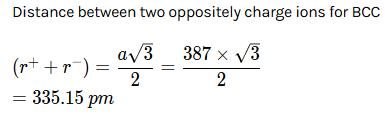QUESTION: 4

The number of atoms contained in a fcc unit cell of a monoatomic substance is

Solution:

The number of atoms contained in one face-centred cubic unit cell of monoatomic substance is 4. Total number of atoms in one fcc unit cell = 1 + 3 = 4

QUESTION: 5

In the fluorite structure, the coordination number of Ca2+ ion is : 

Solution:

In fluorite structure each Ca2+ ion is surrounded by eight F" ions. Thus, the coordination number of Ca2+ is eight.

QUESTION: 6

On doping Ge metal with a little of In or Ga, one gets

Solution:

p-type of semiconductors are produced (a)  due to metal deficiency defects (b)by adding impurity containing less electrons (i.e. atoms of group 13) Ge belongs to Group 14 and In to Group 13.
Hence on doping, p-type semicondutor is obtained.

QUESTION: 7

In graphite electrons are : 

Solution:

In graphite, the electrons are spread out between the sheets.

QUESTION: 8

The pure crystalline substance on being heated gradually first forms a turbid liquid at constant temperature and still at higher temperature turbidity completely disappears. The behaviour is a characteristic of substance forming. 

Solution:

Liquid crystals are matter in a state that has properties between those of conventional liquid and those of solid crystal. For instance, a liquid crystal may flow like a liquid but its molecules may be oriented in a crystal-like way.

QUESTION: 9

When electrons are trapped into the crystal in anion vacancy, the defect is known as : 

Solution:

When electrons are trapped in an ion vacancies, these are called F-centres.

The lattice sites containing the electrons trapped in the anion vacancies are called F-centres because they are responsible for imparting colour to the crystals (F=Farbe which is a German word for colour).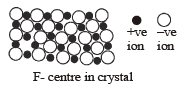QUESTION: 10

A solid with high electrical and thermal conductivity from the following is 

Solution:

Out of the given substances, only Li has high electrical and thermal conductivity.

Li being an alkali metal has high electrical and thermal conductivity due to the presence of one electron in the outermost orbit which is readily available for delocalisation and contribution.

QUESTION: 11

The edge length of a face centered cubic cell of an ionic substance is 508 pm .If the radius of the carbon is 110pm , the radius of the anion is

Solution:

For an fcc solid, edge length , a = 2(r++ r)

⇒ 2(110+r) = 508

⇒ 220+2r− = 508

⇒ 2r= 508−220

⇒2r− = 288

∴ r− = 144pm

QUESTION: 12

The intermetallic compound LiAg crystallizes in a cubic lattice in which both lithium and silver atoms have coordination number of eight. To what crystal class does the unit cell belong ?

Solution:

A body-centred cubic system consists of all eight corners plus one atom at the centre of cube.

QUESTION: 13

For two ionic solids CaO and KI, identify the wrong statement amongst the following : 

Solution:

KI is an ionic compound (polar compound), so, it won't be soluble in a non-polar solvent. Lattice energy is directly proportional to the product of charge and CaO has more charge compared to KI. Lattice energy leads to a high melting point.

QUESTION: 14

Schottky defect in crystals is observed when 

Solution:

If in an ionic crystal of the type A+, B, equal number of cations and anions are missing from their lattice sites so that the electrical neutrality is maintained. The defect is called Schottky defect.

QUESTION: 15

The second order Bragg diffraction of X-rays with = 1.00 Å from a set of parallel planes in a metal occurs at an angle 60º. The distance between the scattering planes in the crystal is

Solution:

Given : Order of Bragg diffraction (n) = 2 ; Wavelength (λ) = 1 Å and angle (θ) = 60º. We know from the Bragg’s equation nλ = 2d sin θ or 2 × 1 = 2d sin 60º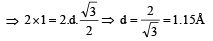(where d = Difference between the scattering planes)

QUESTION: 16

In the solid state, MgO has the same structure as that of sodium chloride. The number of oxygens surrounding each magnesium in MgO is

Solution:

Since MgO has a rock salt structure. In this structure  each cation is surrounded by six anions and vice  versa.

QUESTION: 17

A compound is formed by elements A and B. The crystalline cubic structure has the A atoms at the corners of the cube and B atoms at the body centre. The simplest formula of the compound is 

Solution:

Given: Atoms are present in the corners of cube = A and atom present at body centre = B. We know that a cubic unit cell has 8 corners. Therefore contribution of each atom at the corner =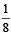Since number of atoms per unit cell is 8, therefore total contribution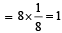We also know that atoms in the body centre, therefore number of atoms per unit cell = 1. Thus formula of the compound is AB.

QUESTION: 18

When molten zinc is converted into solid state, it acquires HCP structure. The number of nearest neighbours will be 

Solution:

hcp is a closed packed arrangement in which the unit cell is hexagonal and coordination number is 12.

QUESTION: 19

The pyknometric den sity of sodium chloride crystal is 2.165 × 103 kg m–3 while its X-ray density is 2.178 × 103 kg m–3. The fraction of unoccupied sites in sodium chloride crystal is

Solution:

Fraction of unoccupied sites in NaCl crystal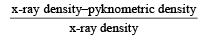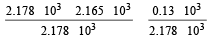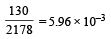QUESTION: 20

A compound for med by elemen ts X an d Y crystallizes in a cubic structure in which the X atoms are at the corners of a cube and the Y atoms are at the face centres. The formula of the compound is 

Solution:

For a cubic structure, No. of  X atoms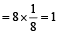No. of Y atoms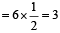∴        Formula of the compound = XY3

QUESTION: 21

In face-centred cubic lattice, a unit cell is shared equally by how many unit cells? 

Solution: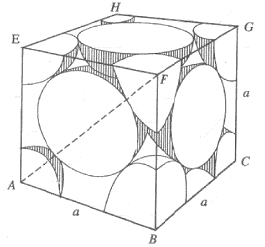An isolated fcc cell is shown here. Each face of the cell is common to two adjacent cells. Therefore, each face centre atom contributes only half of its volume and mass to one cell. Arranging six cells each sharing the remaining half of the face centred atoms, constitutes fcc cubic lattice. e.g., Cu and Al.

QUESTION: 22

The appearance of colour in solid alkali metal halides is generally due to 

Solution:

The appearance of colour in solid alkali metal halide is due to presence of F-centres found as defect in the crystal structure.

QUESTION: 23

CsBr crystallises in a body centered cubic lattice.The unit cell length is 436.6 pm. Given that the atomic mass of Cs = 133 and that of Br = 80 amu and Avogadro number being 6.02 × 1023 mol–1, the density of CsBr is



Solution: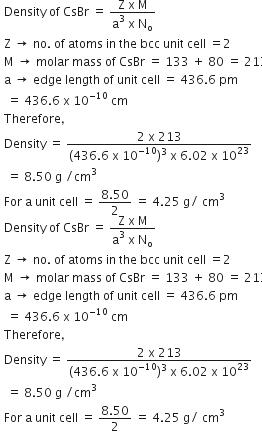QUESTION: 24

If NaCl is doped with 10– 4 mol % of SrCl2, the concentration of cation vacancies will be (NA = 6.02 × 1023 mol–1) 

Solution:

Since each Sr ++ ion pr ovides on e cation vacancy, hence
Concentration of cation vacancies = mole % of SrCl2 added = 10–4 mole %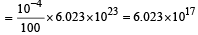QUESTION: 25

Which of the folloiwng anions is present in the chain structure of silicates? 

Solution:

(SiO32–)n Two oxygen atoms per SiO4 tetrahedron are shared giving polymeric anion chains.
Spodumene LiAl (SiO3)2, Diposide CaMg(SiO3)2.

QUESTION: 26

The fraction of total volume occupied by the atoms present in a simple cube is



Solution:

Number of atoms per unit cell = 1 Atoms touch each other along edges. Hence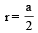( r = radius of atom and a = edge length)

Therefore % fraction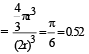QUESTION: 27

If ‘a’ stands for the edge length of the cubic systems : simple cubic, body centred cubic and face centred cubic, then the ratio of radii of the spheres in these systems will be respectively,

Solution:

Following generalization can be easily derived for various types of lattice arrangements in cubic cells between the edge length (a) of the cell and r the radius of the sphere.
For simple cubic :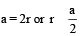For body centred cubic :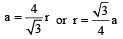For face centred cubic :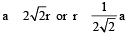Thus the ratio of radii of spheres for these will be simple : bcc : fcc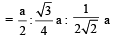i.e. option (a) is correct answer.

QUESTION: 28

Percentage of free space in a body centred cubic unit cell is : 

Solution: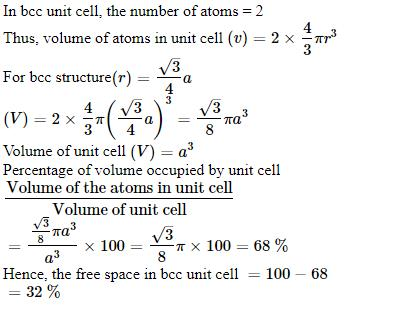QUESTION: 29

With which one of the following elements silicon should be doped so as to give p-type of semiconductor ? 

Solution:

The semiconductors  formed by the introduction of impurity atoms containing one elecron less than the parent atoms of insulators are termed as p-type semiconductors. Therefore silicon containing 14 electrons is to be doped with boron containing 13 electrons to give a p- type semi-conductor.

QUESTION: 30

Lithium metal crystallises in a body centred cubic crystal. If the length of the side of the unit cell of lithium is 351 pm, the atomic radius of the lithium will be:

Solution:

Since lithium metal crystallises in a body centred cubic crystal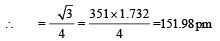QUESTION: 31

Copper crystallises in a face-centred cubic lattice with a unit cell length of 361 pm. What is the radius of copper atom in pm? 

Solution:

Since Cu  metal crystallises in a face centred cubic lattice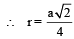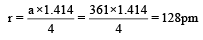QUESTION: 32

AB; crystallizes in a body centred cubic lattice with edge length ‘ ’ equal to 387 pm . The distance between two oppositely charged ions in the lattice is : 

Solution:

For BCC lattice body diagonal =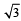The distance between the two oppositely charged ions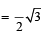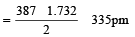QUESTION: 33

Among the following which one has the highest cation to anion size ratio? 

Solution: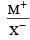is highest in CsF

∴    Correct choice : (c)

QUESTION: 34

A solid compound XY has NaCl structure. If the radius of the cation is 100 pm, the radius of the anion (Y) will be : [2011 M]

Solution:

Radius ratio of NaCl like crystal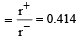=100/0.414= 241.5= r-

QUESTION: 35

A metal crystallizes with a face-centered cubic lattice. The edge length of the unit cell is 408 pm.The diameter of the metal atom is : 

Solution:

For fcc lattice,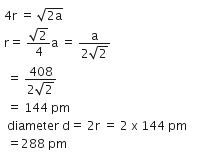QUESTION: 36

The number of octahedral void(s) per atom present in a cubic close-packed structure is : 

Solution:

Number of octahedral voids in ccp, is equal to effective number of atoms.
In ccp, effective number of atoms are 4 so, 4 octahedral voids.
So, 1 octahedral voids per atom.

QUESTION: 37

Structure of a mixed oxide is cubic close-packed (c.c.p). The cubic unit cell of mixed oxide is composed of oxide ions. One fourth of the tetrahedral voids are occupied by divalent metal A and the octahedral voids are occupied by a monovalent metal B. The formula of the oxide is : [2012 M]

Solution: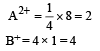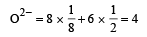Hence the formula of the oxide will be A2B4O4 or AB2O2.

QUESTION: 38

A metal has a fcc lattice. The edge length of the unit cell is 404 pm. The density of the metal is 2.72 g cm-3.The molar mass of the metal is?

(NA Avogadro's constant= 6.02 x 1023 mol-1) [NEET 2013]

Solution:

Given, cell is fcc, So Z =4
Edge length, a = 404 pm = 4.04 x 10-8 cm
Density of metal, d = 2.72 g cm-3
NA = 6.02 x 1023 mol-1
Molar mass ofg the metal, M =?
We know that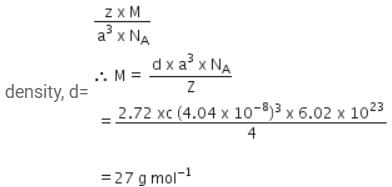QUESTION: 39

The number of carbon atoms per unit cell of diamond unit cell is : [NEET 2013]

Solution:

Diamond is like ZnS. In diamond cubic unit cell, there are eight corner atoms, six face centered atoms and four more atoms inside the structure.
Number of atoms present in a diamond cubic cell

=8*1/8 + 6*1/2 +4 = 8